Research website of Vyacheslav Gorchilin
2021-05-03
Vector of the second magnetic field
Authors: Gorchilin V.V., Dmitry S.
My co-author, Dmitry, has been engaged in practical research and the problem of vector magnetic fields for quite some time. For a long time, some of the energy relationships did not converge for him, if they are counted according to the classical formulas. Non-classical winding of coils, for example with a caduceus, did not even come close to calculating the observed result. Practice pushed the theory to create a mathematical apparatus that would complement the classics and correspond to experimental data. This is the math we present here.
As you know, if there are two vectors located at an angle to each other, then they will add up according to the vector addition rule (Fig. 1): $\vec B_I = \vec B_1 + \vec B_2 \qquad (1)$ Here: $$\vec B_1, \vec B_2$$ - forming vectors of magnetic induction from which the resulting vector $$\vec B_I$$ is obtained. This vector will be called the induction vector of the first (classical) magnetic field (MF).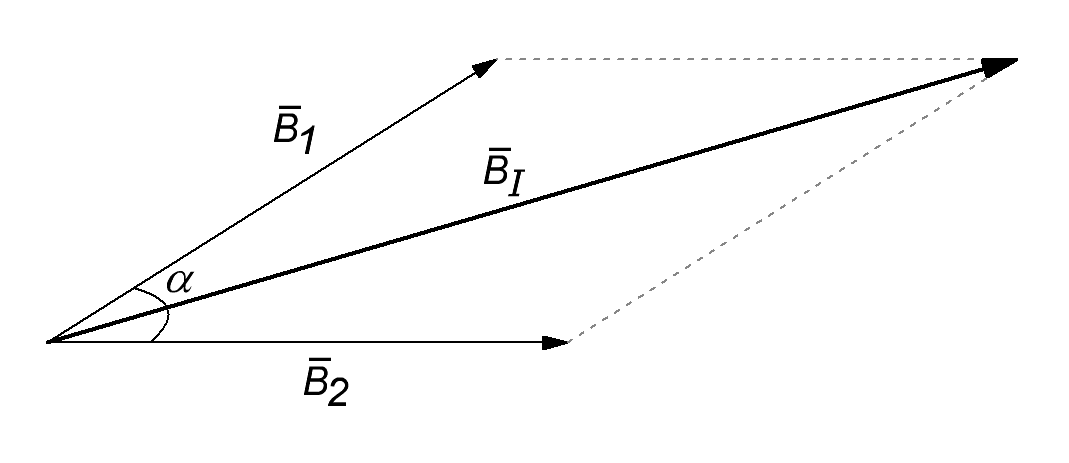Fig.1. Addition of two vectors of magnetic induction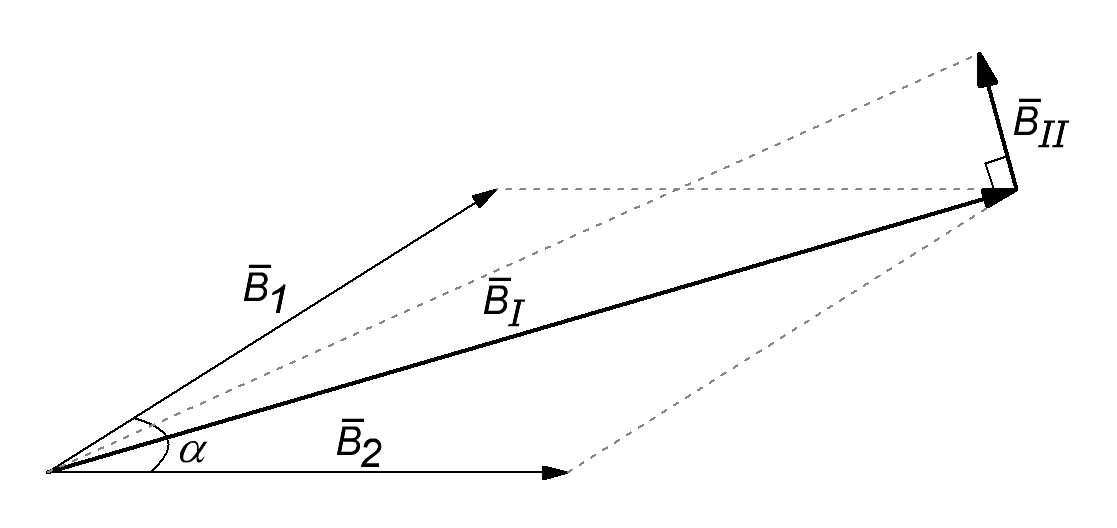Fig.2. Addition of vectors taking into account the second MF
Further, for simplicity of display, we will denote the lengths of vectors as follows: $| \vec B_I | = B_I, \quad | \vec B_ {II} | = B_ {II}, \quad | \vec B_1 | = B_1, \quad | \vec B_2 | = B_2$ Using these designations, we find the square of the vector length of the first MF by multiplying expression (1) by itself : $B_I ^ 2 = B_1 ^ 2 + B_2 ^ 2 + 2 B_1 B_2 \cos (\alpha) \qquad (2)$ Here: $$\alpha$$ is the angle between the forming vectors. This apparatus offers the classical theory, but without taking into account the second magnetic field, it turns out to be incomplete.
Based on experiments and observations, it is proposed to take into account the second MF as follows: $B_ {II} ^ 2 = B_I (\alpha = 0) ^ 2 - B_I ^ 2 \qquad (3)$ where on the right side of the expression, the first term is obtained at a zero angle between the forming vectors, and $$B_ {II}$$ is the length of the vector of the second MF.
The direction of the induction vector of the second MF is shown in Figure 2. This vector must be perpendicular to the plane of the drawing, but since further we will consider only its length, then it is the length of this vector that will be reflected in the figures, and its direction and position are rather arbitrary.
From here (2,3) we derive the value of the square of the length of the induction vector of the second MF: $B_ {II} ^ 2 = B_1 ^ 2 + B_2 ^ 2 + 2 B_1 B_2 - B_I ^ 2 = 4 B_1 B_2 \sin ^ 2 (\alpha / 2) \qquad (4)$ In the last formula, you need to pay attention to the multiplication factor "4" in front of the sinus. It can be extremely important for free energy seekers. Also, from the last formula, you can derive an interesting energy ratio $B_I ^ 2 + B_ {II} ^ 2 = (B_1 + B_2) ^ 2 \qquad (5)$ from which, in turn, the following rule is derived:

The sum of the squares of the lengths of the vectors of the first and second MF is always equal to the square of the sum of the lengths of the forming vectors.

The following figures show examples of vectors of the first and second MF depending on the angle between the forming vectors.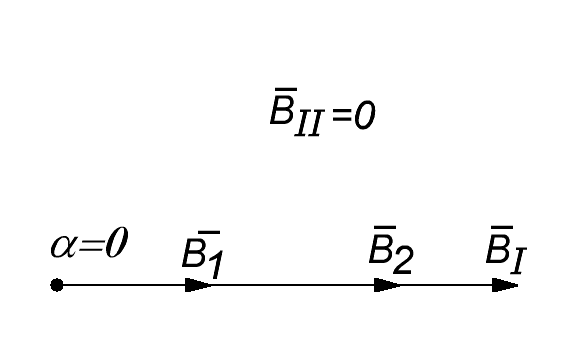Fig.3. α = 0°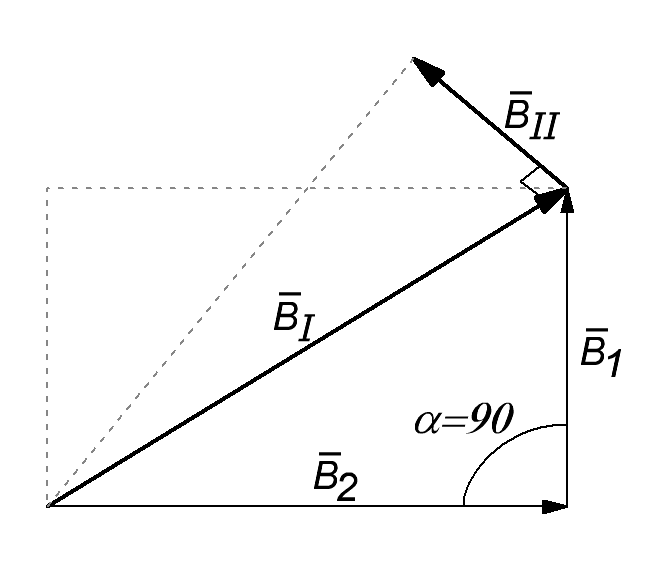Fig.4. α = 90°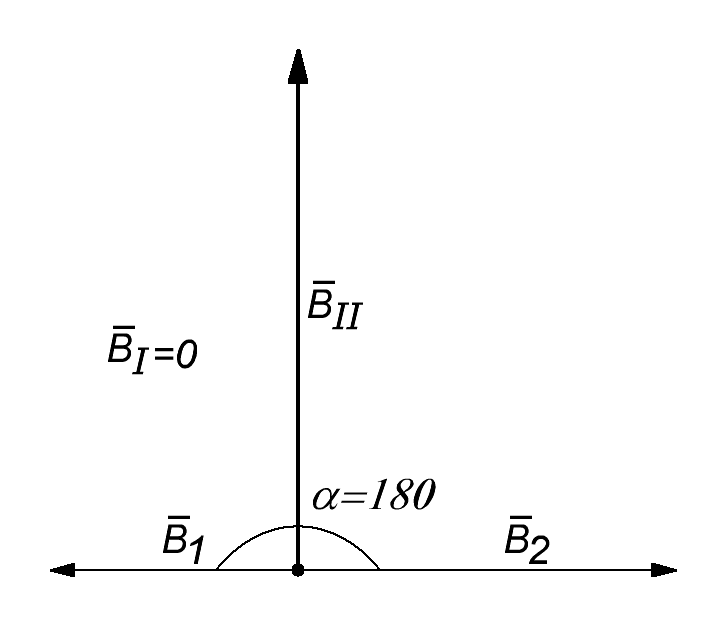Fig.5. α = 180°
Complementing Maxwell
Now we can supplement Maxwell in terms of the specific energy of the electromagnetic field , with regard to its magnetic component. To do this, simply multiply formula (5): $w = {1 \over 2 \mu_0 \mu} \left (B_I ^ 2 + B_ {II} ^ 2 \right) = {1 \over 2 \mu_0 \mu} (B_1 + B_2) ^ 2 \qquad (6)$ Where: $$\mu \mu_0$$ - relative and constant magnetic permeabilities, and $$w$$ is the specific energy of the magnetic component of the electromagnetic field.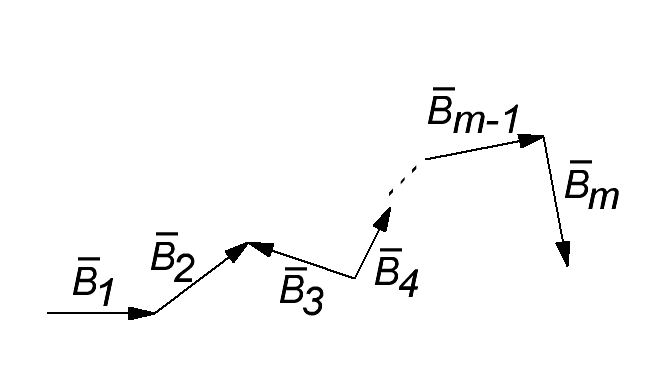Fig.6. Sum of m vectors
Obviously, for several forming vectors, formula (6) will be written in a more general form as follows: $w = {1 \over 2 \mu_0 \mu} \left (B_I ^ 2 + B_ {II} ^ 2 \right) = {1 \over 2 \mu_0 \mu} \left (\sum ^ m B_n \right) ^ 2 \qquad (7)$ Where $$B_n$$ is the n-th forming vector of magnetic induction, the total number of which is $$m$$. All vectors must be connected in series (Fig. 6).
Hence the following conclusion is drawn:

The energy of the magnetic component (including the first and second MF) of the electromagnetic field does not depend on the angle between the forming vectors.

The authors suggest that such a conclusion is more energetically correct than that of the classic, for whom this energy depends on this angle. Now we can talk about a superposition of vectors, the total energy of which does not depend on their relative position.
With the equality of the inductions of the first and second MF, for two forming vectors (6), a rather interesting analytical formula is also obtained: $w = {1 \over \mu_0 \mu} B ^ 2, \quad B_ {I} = B_ {II} = B = {B_1 + B_2 \over \sqrt {2}} \qquad (8)$ In these expressions, $$w$$ reflects the total specific energy of the first and second MF. The authors see that similar calculations can be made for the electrical component of the total energy of the electromagnetic field , but as an analogue of the second MF there it will be necessary to use the displacement current and vector potential.
Illustrate vectors
Dmitry in the video shows completely unusual experiments from the point of view of classical magnetic fields. The magnetic field in the torus must be closed inside it and the light bulb must not glow. It is impossible to explain these experiments without vectors perpendicular to the first magnetic field.
In the following videos, the researcher Segey Deyna clearly demonstrates the presence of the second MP, and its orientation in relation to the forming vectors. In this case, the direction of the second MF implies a certain plane of vectors perpendicular to the vectors of the first MF.
Live view of vectors
Below is a simple calculator, which, based on the above formulas (2-4), allows you to calculate the lengths of the vectors of the first and second MF ($$B_I, B_ {II}$$) depending on the relative position and lengths of the two forming vectors $$B_1, B_2$$. It should be recalled that the vector of the second MF is always perpendicular to the plane of the forming vectors, and to the vector of the first MF.
Vector length $$B_1$$
Vector length $$B_2$$
Angle between vectors, degrees
Vector length of the first MF $$B_I$$
1
Vector length of the second MF $$B_ {II}$$
1
Materials used
1. Wikipedia. Scalar product of vectors.
2. Wikipedia. Energy electromagnetic field.